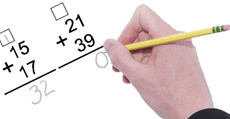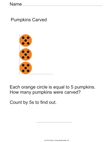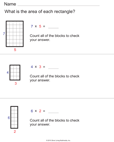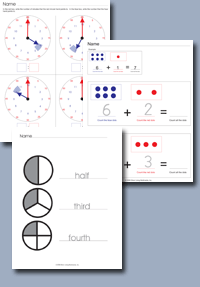﻿ Common Core Standards - Math - 3rd GradeAdaptive Worksheets

Helping Every Child to Succeed

toll-free (888) 777-0876
fax (888) 777-0875

## Solve Problems Involving Measurement and Estimation.

• 3.MD.A.1. Tell and write time to the nearest minute and measure time intervals in minutes. Solve word problems involving addition and subtraction of time intervals in minutes, e.g., by representing the problem on a number line diagram.
• 3.MD.A.2. Measure and estimate liquid volumes and masses of objects using standard units of grams (g), kilograms (kg), and liters (l).1 Add, subtract, multiply, or divide to solve one-step word problems involving masses or volumes that are given in the same units, e.g., by using drawings (such as a beaker with a measurement scale) to represent the problem.

## Represent and Interpret Data.

• 3.MD.B.3. Draw a scaled picture graph and a scaled bar graph to represent a data set with several categories. Solve one- and two-step “how many more” and “how many less” problems using information presented in scaled bar graphs. For example, draw a bar graph in which each square in the bar graph might represent 5 pets.Scaled Pumpkin Bar Graph with Hints
• 3.MD.B.4 Generate measurement data by measuring lengths using rulers marked with halves and fourths of an inch. Show the data by making a line plot, where the horizontal scale is marked off in appropriate units— whole numbers, halves, or quarters.Measure Lines and Plot

## Geometric measurement: understand concepts of area and relate area to multiplication and to addition.

• 3.MD.C.5 Recognize area as an attribute of plane figures and understand concepts of area measurement.
• MD.C.5a A square with side length 1 unit, called “a unit square,” is said to have “one square unit” of area, and can be used to measure area.
• MD.C.5b A plane figure which can be covered without gaps or overlaps by n unit squares is said to have an area of n square units.
• 3.MD.C.6 Measure areas by counting unit squares (square cm, square m, square in, square ft, and improvised units).
• C.7 Relate area to the operations of multiplication and addition.
• 3.MD.C.7a Find the area of a rectangle with whole-number side lengths by tiling it, and show that the area is the same as would be found by multiplying the side lengths.• 3.MD.C.7b Multiply side lengths to find areas of rectangles with whole-number side lengths in the context of solving real world and mathematical problems, and represent whole-number products as rectangular areas in mathematical reasoning.
• 3.MD.C.7c Use tiling to show in a concrete case that the area of a rectangle with whole-number side lengths a and b + c is the sum of a × b and a × c. Use area models to represent the distributive property in mathematical reasoning.
• 3.MD.C.7d Recognize area as additive. Find areas of rectilinear figures by decomposing them into non-overlapping rectangles and adding the areas of the non-overlapping parts, applying this technique to solve real world problems.

## Geometric measurement: understand concepts of area and relate area to multiplication and to addition.

• 3.MD.D.8 Solve real world and mathematical problems involving perimeters of polygons, including finding the perimeter given the side lengths, finding an unknown side length, and exhibiting rectangles with the same perimeter and different areas or with the same area and different perimeters.